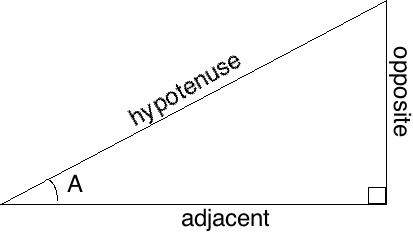# MPM2D Grade 10 Math Trigonometry Test

Trigonometric Identities

• Tangent
• Sine
• Cosine

MEMORIZE: SOH CAH TOASine = opposite/hypotenuse

*For Theta angles, use the inverse with Shift + (trig ratio)*

*Make sure calculator is in Degree mode before continuing test/exam*

Sine Law

• SinA/a = SinB/b = SinC/c

Video on how to apply Sin Law

Cosine Law
a^2 = b^2+c^2-2(b)(c)cosA
b^2 = c^2+a^2-2(c)(a)cosB
c^2 = b^2+a^2-2(b)(a)cosC

Video on how to apply the Cosine Law